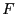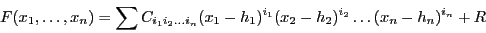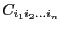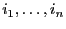Next: Miscellaneous procedures Up: Definite integrals Previous: Integral with one variable   Contents

Integral with multiple variable

ALIAS offers 4 procedures to compute definite integral with more than one variable.

int IntegrateMultiRectangle(
INTERVAL_VECTOR (* Function)
(int,int,INTERVAL_VECTOR &),
INTERVAL_VECTOR (* Second_Derivative)
(int,int,INTERVAL_VECTOR &),
INTERVAL_VECTOR & TheDomain,
int Iteration,
double Accuracy,
INTERVAL & Result)

int IntegrateMultiRectangle(
INTERVAL_VECTOR (* Function)
(int,int,INTERVAL_VECTOR &),
INTERVAL_VECTOR (* Second_Derivative)
(int,int,INTERVAL_VECTOR &),
INTERVAL_VECTOR & TheDomain,
int Iteration,
double Accuracy,
INTERVAL & Result)
In the second form Gradient is a procedure in MakeJ format that allows to compute the derivatives of the second derivatives of the function. ALIAS offer also procedure based on Taylor expansion of the function.

int IntegrateMultiTaylor(
INTERVAL_VECTOR (* CoeffInt)
(int,int,INTERVAL_VECTOR &),
INTERVAL_VECTOR (* RestTaylor)
(int,int,INTERVAL_VECTOR &),
INTEGER_MATRIX &APOWERINT,
INTEGER_MATRIX &APOWERREM,
int nbrem,
int Order,
INTERVAL_VECTOR & TheDomain,
int Iteration,
double Accuracy,
INTERVAL & Result)
int IntegrateMultiTaylor(
INTERVAL_VECTOR (* CoeffInt)
(int,int,INTERVAL_VECTOR &),
INTERVAL_VECTOR (* RestTaylor)
(int,int,INTERVAL_VECTOR &),
INTEGER_MATRIX &APOWERINT,
int Order,
INTERVAL_VECTOR & TheDomain,
int Iteration,
double Accuracy,
INTERVAL & Result)
The Taylor expansion of the functionmay be written as:• CoeffInt: a procedure in MakeF format that compute the coefficients• APOWERINT: a table with the exponentfor each term
• RestTaylor, APOWERREM: in the first form they play the same role than CoeffInt, APOWERINT for the remainder. There is nbrem terms in the remainder
• RestTaylor: in the second form a procedure in MakeF format that compute the remainder
• Order: the degree of the remainder

Jean-Pierre Merlet 2012-12-20